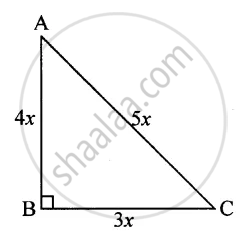# The Lengths of the Sides of a Triangle Are in the Ratio 4 : 5 : 3 and Its Perimeter is 96 Cm. Find Its Area. - Mathematics

Sum

The lengths of the sides of a triangle are in the ratio 4: 5 : 3 and its perimeter is 96 cm. Find its area.

#### Solution

Let the sides of the triangle ABC be 4x, 5x and 3x
Let AB = 4x, AC = 5x and BC = 3x
Perimeter = 4x + 5x + 3x = 96
=> 12x = 96

⇒ x = 96/12∴ x = 8

∴ Sides are

BC = 3(8) = 24 cm, AB = 4(8) = 32 cm,

AC = 5(8) = 40 cm

Since (AC)2 = (AB)2 + (BC)2   [∵ (5x)2 = (3x)2 + (4x)2]

∴ By Pythagorus Theorem, ∠B = 90°

∴ Area of ΔABC = 1/2 ("BC")("AB") = 1/2 (24)(32)

= 12 xx 32 = 384  "cm"^2

Is there an error in this question or solution?

#### APPEARS IN

Selina Concise Mathematics Class 8 ICSE
Chapter 20 Area of a Trapezium and a Polygon
Exercise 20 (A) | Q 10 | Page 224